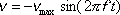This is the equation for the maximum velocity of an object undergoing simple harmonic motion.  The object has maximum velocity when the object is at the equilibrium position.  As you can see, the maximum velocity of the object depends on A the amplitude of the oscillations and f the frequency of the oscillations.

Note:  This simple little equation can be crucial when you need to use the equation,  which, without a value for vmax may not be at all helpful.